SBAS627 July   2015

PRODUCTION DATA.

1. Features
2. Applications
3. Description
4. Revision History
5. Device Comparison Table
6. Pin Configuration and Functions
7. Specifications
8. Detailed Description
1. 8.1 Overview
2. 8.2 Functional Block Diagram
3. 8.3 Feature Description
4. 8.4 Device Functional Modes
1. 8.4.1 Device Interface
1. 8.4.1.1 Digital Pin Description
2. 8.4.1.2 Data Acquisition Example
3. 8.4.1.3 Host-to-Device Connection Topologies
2. 8.4.2 Device Modes
5. 8.5 Register Maps
1. 8.5.1 Command Register Description
2. 8.5.2 Program Register Description
9. Application and Implementation
1. 9.1 Application Information
2. 9.2 Typical Applications
10. 10Power-Supply Recommendations
11. 11Layout
12. 12Device and Documentation Support
13. 13Mechanical, Packaging, and Orderable Information

• DBT|38

## 7 Specifications

### 7.1 Absolute Maximum Ratings

over operating free-air temperature range (unless otherwise noted)(1)
MIN MAX UNIT
AIN_nP, AIN_nGND to GND(2) –20 20 V
AIN_nP, AIN_nGND to GND(3) –11 11 V
AUX_GND to GND –0.3 0.3 V
AUX_IN to GND –0.3 AVDD + 0.3 V
AVDD to GND or DVDD to GND –0.3 7 V
REFCAP to REFGND or REFIO to REFGND –0.3 5.7 V
GND to REFGND –0.3 0.3 V
Digital input pins to GND –0.3 DVDD + 0.3 V
Digital output pins to GND –0.3 DVDD + 0.3 V
Operating temperature, TA –40 125 °C
Storage temperature, Tstg –65 150 °C
(1) Stresses beyond those listed under Absolute Maximum Ratings may cause permanent damage to the device. These are stress ratings only, and do not imply functional operation of the device at these or any other conditions beyond those indicated under Recommended Operating Conditions. Exposure to absolute-maximum-rated conditions for extended periods may affect device reliability.
(2) AVDD = 5 V or offers a low impedance of < 30 kΩ.
(3) AVDD = floating with an impedance > 30 kΩ.

### 7.2 ESD Ratings

VALUE UNIT
V(ESD) Electrostatic discharge Human body model (HBM), per ANSI/ESDA/JEDEC JS-001(1) Analog input pins (AIN_nP; AIN_nGND) ±4000 V
All other pins ±2000
Charged device model (CDM), per JEDEC specification JESD22-C101(2) ±500
(1) JEDEC document JEP155 states that 500-V HBM allows safe manufacturing with a standard ESD control process.
(2) JEDEC document JEP157 states that 250-V CDM allows safe manufacturing with a standard ESD control process.

### 7.3 Recommended Operating Conditions

over operating free-air temperature range (unless otherwise noted)
MIN NOM MAX UNIT
AVDD Analog supply voltage 4.75 5 5.25 V
DVDD Digital supply voltage 1.65 3.3 AVDD V

### 7.4 Thermal Information

DBT (TSSOP)
38 PINS
RθJA Junction-to-ambient thermal resistance 68.8 °C/W
RθJC(top) Junction-to-case (top) thermal resistance 19.9 °C/W
RθJB Junction-to-board thermal resistance 30.4 °C/W
ψJT Junction-to-top characterization parameter 1.3 °C/W
ψJB Junction-to-board characterization parameter 29.8 °C/W
RθJC(bot) Junction-to-case (bottom) thermal resistance NA °C/W

### 7.5 Electrical Characteristics

Minimum and maximum specifications are at TA = –40°C to 125°C. Typical specifications are at TA = 25°C.
AVDD = 5 V, DVDD = 3 V, VREF = 4.096 V (internal), and fSAMPLE = 500 kSPS, unless otherwise noted.
PARAMETER TEST CONDITIONS MIN TYP MAX UNIT TEST LEVEL(1)
Full-scale input span(2)
(AIN_nP to AIN_nGND)
Input range = ±2.5 × VREF –2.5 × VREF 2.5 × VREF V A
Input range = ±1.25 × VREF –1.25 × VREF 1.25 × VREF A
Input range = ±0.625 × VREF –0.625 × VREF 0.625 × VREF A
Input range = ±0.3125 × VREF –0.3125 × VREF 0.3125 × VREF A
Input range = ±0.15625 × VREF –0.15625 × VREF 0.15625 × VREF A
Input range = 2.5 × VREF 0 2.5 × VREF A
Input range = 1.25 × VREF 0 1.25 × VREF A
Input range = 0.625 × VREF 0 0.625 × VREF A
Input range = 0.3125 × VREF 0 0.3125 × VREF A
AIN_nP Operating input range,
positive input
Input range = ±2.5 × VREF –2.5 × VREF 2.5 × VREF V A
Input range = ±1.25 × VREF –1.25 × VREF 1.25 × VREF A
Input range = ±0.625 × VREF –0.625 × VREF 0.625 × VREF A
Input range = ±0.3125 × VREF –0.3125 × VREF 0.3125 × VREF A
Input range = ±0.15625 × VREF –0.15625 × VREF 0.15625 × VREF A
Input range = 2.5 × VREF 0 2.5 × VREF A
Input range = 1.25 × VREF 0 1.25 × VREF A
Input range = 0.625 × VREF 0 0.625 × VREF A
Input range = 0.3125 × VREF 0 0.3125 × VREF A
AIN_nGND Operating input range,
negative input
All input ranges –0.1 0 0.1 V B
zi Input impedance At TA = 25°C,
all input ranges
0.85 1 1.15 B
Input impedance drift All input ranges 7 25 ppm/°C B
IIkg(in) Input leakage current With voltage at AIN_nP pin = VIN,
input range = ±2.5 × VREF
VIN – 2.25
————
RIN
µA A
With voltage at AIN_nP pin = VIN,
input range = ±1.25 × VREF
VIN – 2.00
————
RIN
A
With voltage at AIN_nP pin = VIN,
input ranges = ±0.625 × VREF; ±0.3125 × VREF; ±0.15625 × VREF
VIN – 1.60
————
RIN
A
With voltage at AIN_nP pin = VIN,
input range = 2.5 × VREF
VIN – 2.50
————
RIN
A
With voltage at AIN_nP pin = VIN,
input range = 1.25 × VREF; 0.625 × VREF; 0.3125 × VREF
VIN – 2.50
————
RIN
A
INPUT OVERVOLTAGE PROTECTION
VOVP Overvoltage protection voltage AVDD = 5 V or offers low impedance < 30 kΩ, all input ranges –20 20 V B
AVDD = floating with impedance
> 30 kΩ, all input ranges
–11 11 B
SYSTEM PERFORMANCE
Resolution 14 Bits A
NMC No missing codes 14 Bits A
DNL Differential nonlinearity –0.5 ±0.2 0.5 LSB(3) A
INL Integral nonlinearity(6) –0.75 ±0.25 0.75 LSB A
EG Gain error At TA = 25°C, all input ranges ±0.02 ±0.05 %FSR(4) A
Gain error matching
(channel-to-channel)
At TA = 25°C, all input ranges ±0.02 ±0.05 %FSR A
Gain error temperature drift All input ranges 1 4 ppm/°C B
EO Offset error At TA = 25°C,
input range = ±2.5 × VREF(10)
±0.5 ±1 mV A
At TA = 25°C,
all other input ranges
±0.5 ±2 A
Offset error matching
(channel-to-channel)
At TA = 25°C,
input range = ±2.5 × VREF(10)
±0.5 ±1 mV A
At TA = 25°C,
all other input ranges
±0.5 ±2 A
Offset error temperature drift Input range = ±2.5 × VREF 1 3 ppm/°C B
Input range = ±1.25 × VREF 1 3 B
Input range = ±0.625 × VREF 1 3 B
Input range = ±0.3125 × VREF 2 6 B
Input range = ±0.15625 × VREF 4 12 B
Input range = 0 to 2.5 × VREF 1 3 B
Input range = 0 to 1.25 × VREF 1 3 B
Input range = 0 to 0.625 × VREF 2 6 B
Input range = 0 to 0.3125 × VREF 4 12 B
SAMPLING DYNAMICS
tCONV Conversion time 850 ns A
tACQ Acquisition time 1150 ns A
fS Maximum throughput rate
without latency
500 kSPS A
DYNAMIC CHARACTERISTICS
SNR Signal-to-noise ratio
(VIN – 0.5 dBFS at 1 kHz)
Input range = ±2.5 × VREF 84.5 85 dB A
Input range = ±1.25 × VREF 84.2 85 A
Input range = ±0.625 × VREF 83.5 84.5 A
Input range = ±0.3125 × VREF 80 82 A
Input range = ±0.15625 × VREF 75 77 A
Input range = 2.5 × VREF 84 85 A
Input range = 1.25 × VREF 83.5 84.5 A
Input range = 0.625 × VREF 80 82 A
Input range = 0.3125 × VREF 75 77 A
THD Total harmonic distortion(5)
(VIN – 0.5 dBFS at 1 kHz)
All input ranges –100 dB B
(VIN – 0.5 dBFS at 1 kHz)
Input range = ±2.5 × VREF 84.3 84.8 dB A
Input range = ±1.25 × VREF 84 84.8 A
Input range = ±0.625 × VREF 83.3 84.3 A
Input range = ±0.3125 × VREF 80 82 A
Input range = ±0.15625 × VREF 75 77 A
Input range = 2.5 × VREF 83.8 84.8 A
Input range = 1.25 × VREF 83.3 84.3 A
Input range = 0.625 × VREF 80 82 A
Input range = 0.3125 × VREF 75 77 A
SFDR Spurious-free dynamic range
(VIN – 0.5 dBFS at 1 kHz)
All input ranges 101 dB B
Crosstalk isolation(7) Aggressor channel input overdriven to 2 × maximum input voltage 110 dB B
Crosstalk memory(8) Aggressor channel input overdriven to 2 × maximum input voltage 90 dB B
BW(–3 dB) Small-signal bandwidth, –3 dB At TA = 25°C, all input ranges 15 kHz B
BW(–0.1 dB) Small-signal bandwidth, –0.1 dB At TA = 25°C, all input ranges 2.5 kHz B
AUXILIARY CHANNEL
Resolution 14 Bits A
V(AUX_IN) AUX_IN voltage range (AUX_IN – AUX_GND) 0 VREF V A
Operating input range AUX_IN 0 VREF V A
AUX_GND 0 V A
Ci Input capacitance During sampling 75 pF C
During conversion 5 pF C
IIkg(in) Input leakage current 100 nA A
DNL Differential nonlinearity –0.75 ±0.5 0.75 LSB A
INL Integral nonlinearity –1 ±0.5 1 LSB A
EG(AUX) Gain error At TA = 25°C ±0.02 ±0.2 %FSR A
EO(AUX) Offset error At TA = 25°C –5 5 mV A
SNR Signal-to-noise ratio V(AUX_IN) = –0.5 dBFS at 1 kHz 83.3 84.4 dB A
THD Total harmonic distortion(5) V(AUX_IN) = –0.5 dBFS at 1 kHz –100 dB B
SINAD Signal-to-noise + distortion V(AUX_IN) = –0.5 dBFS at 1 kHz 83 84.3 dB A
SFDR Spurious-free dynamic range V(AUX_IN) = –0.5 dBFS at 1 kHz 100 dB B
INTERNAL REFERENCE OUTPUT
V(REFIO_INT)(9) Voltage on REFIO pin
(configured as output)
At TA = 25°C 4.094 4.096 4.098 V A
Internal reference temperature drift 8 20 ppm/°C B
C(OUT_REFIO) Decoupling capacitor on REFIO 10 22 µF B
(on REFCAP pin)
At TA = 25°C 4.094 4.096 4.098 V A
Reference buffer output impedance 0.5 1 Ω B
Reference buffer temperature drift 0.6 1.5 ppm/°C B
C(OUT_REFCAP) Decoupling capacitor on REFCAP 10 22 μF B
Turn-on time C(OUT_REFCAP) = 22 µF,
C(OUT_REFIO) = 22 µF
15 ms B
EXTERNAL REFERENCE INPUT
VREFIO_EXT External reference voltage on REFIO (configured as input) 4.046 4.096 4.146 V C
POWER-SUPPLY REQUIREMENTS
AVDD Analog power-supply voltage Analog supply 4.75 5 5.25 V B
DVDD Digital power-supply voltage Digital supply range 1.65 3.3 AVDD V B
Digital supply range for specified performance 2.7 3.3 5.25 B
IAVDD_DYN Analog supply current Dynamic, AVDD For the ADS8678; AVDD = 5 V, fS = maximum and internal reference 13 16 mA A
For the ADS8674; AVDD = 5 V, fS = maximum and internal reference 8.5 11.5 A
IAVDD_STC Static For the ADS8678; AVDD = 5 V, device not converting and internal reference 10 12 mA A
For the ADS8674; AVDD = 5 V, device not converting and internal reference 5.5 8.5 A
ISTDBY Standby At AVDD = 5 V, device in STDBY mode and internal reference 3 4.5 mA A
IPWR_DN Power-down At AVDD = 5 V, device in PWR_DN 3 20 μA B
IDVDD_DYN Digital supply current At DVDD = 3.3 V, output = 0000h 0.5 mA A
DIGITAL INPUTS (CMOS)
VIH Digital input logic levels
DVDD > 2.1 V
0.7 × DVDD DVDD + 0.3 V A
VIL –0.3 0.3 × DVDD A
VIH Digital input logic levels
DVDD ≤ 2.1 V
0.8 × DVDD DVDD + 0.3 V A
VIL –0.3 0.2 × DVDD A
Input leakage current 100 nA A
Input pin capacitance 5 pF C
DIGITAL OUTPUTS (CMOS)
VOH Digital output logic levels IO = 500-μA source 0.8 × DVDD DVDD V A
VOL IO = 500-μA sink 0 0.2 × DVDD A
Floating state leakage current Only for SDO 1 µA A
Internal pin capacitance 5 pF C
TEMPERATURE RANGE
TA Operating free-air temperature –40 125 °C B
(1) Test Levels: (A) Tested at final test. Over temperature limits are set by characterization and simulation. (B) Limits set by characterization and simulation, across temperature range. (C) Typical value only for information, provided by design simulation.
(2) Ideal input span, does not include gain or offset error.
(3) LSB = least significant bit.
(4) FSR = full-scale range.
(5) Calculated on the first nine harmonics of the input frequency.
(6) This parameter is the endpoint INL, not best-fit INL.
(7) Isolation crosstalk is measured by applying a full-scale sinusoidal signal up to 10 kHz to a channel, not selected in the multiplexing sequence, and measuring its effect on the output of any selected channel.
(8) Memory crosstalk is measured by applying a full-scale sinusoidal signal up to 10 kHz to a channel that is selected in the multiplexing sequence, and measuring its effect on the output of the next selected channel for all combinations of input channels.
(9) Does not include the variation in voltage resulting from solder-shift and long-term effects.
(10) Does not include the shift in offset over time.

### 7.6 Timing Requirements: Serial Interface

Minimum and maximum specifications are at TA = –40°C to 125°C. Typical specifications are at TA = 25°C.
AVDD = 5 V, DVDD = 3 V, VREF = 4.096 V (internal), SDO load = 20 pF, and fSAMPLE = 500 kSPS, unless otherwise noted.
MIN TYP MAX UNIT
TIMING SPECIFICATIONS
fS Sampling frequency (fCLK = max) 500 kSPS
tS ADC cycle time period (fCLK = max) 2 µs
fSCLK Serial clock frequency (fS = max) 17 MHz
tSCLK Serial clock time period (fS = max) 59 ns
tCONV Conversion time 850 ns
tDZ_CSDO Delay time: CS falling to data enable 10 ns
tD_CKCS Delay time: last SCLK falling to CS rising 10 ns
tDZ_CSDO Delay time: CS rising to SDO going to 3-state 10 ns
TIMING REQUIREMENTS
tACQ Acquisition time 1150 ns
tPH_CK Clock high time 0.4 0.6 tSCLK
tPL_CK Clock low time 0.4 0.6 tSCLK
tPH_CS CS high time 30 ns
tSU_CSCK Setup time: CS falling to SCLK falling 30 ns
tHT_CKDO Hold time: SCLK falling to (previous) data valid on SDO 10 ns
tSU_DOCK Setup time: SDO data valid to SCLK falling 25 ns
tSU_DICK Setup time: SDI data valid to SCLK falling 5 ns
tHT_CKDI Hold time: SCLK falling to (previous) data valid on SDI 5 ns
tSU_DSYCK Setup time: DAISY data valid to SCLK falling 5 ns
tHT_CKDSY Hold time: SCLK falling to (previous) data valid on DAISY 5 nsFigure 1. Serial Interface Timing Diagram

### 7.7 Typical Characteristics

At TA = 25°C, AVDD = 5 V, DVDD = 3 V, internal reference VREF = 4.096 V, and fSAMPLE = 500 kSPS, unless otherwise noted.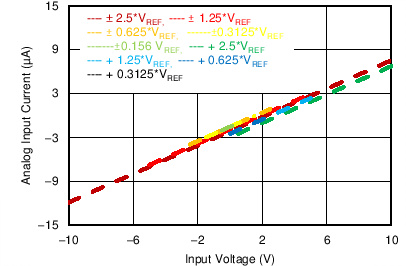Figure 2. Input I-V Characteristic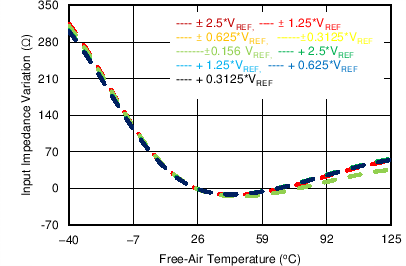Figure 4. Input Impedance Variation vs Temperature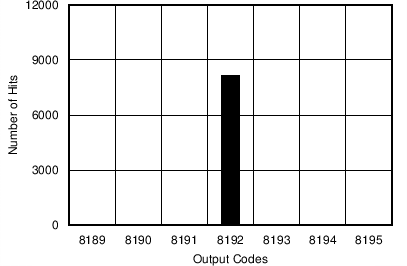Mean = 8192.5, sigma = 0.31, input = 0 V, range = ±2.5 × VREF
Figure 6. DC Histogram for Mid-Scale Inputs (±2.5 × VREF)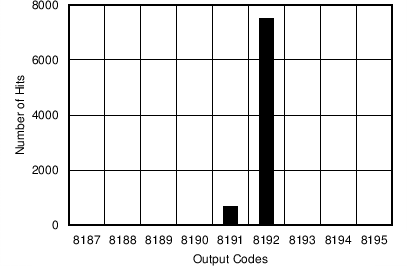Mean = 8192.5, sigma = 0.34, input = 0 V, range = ±0.625 × VREF
Figure 8. DC Histogram for Mid-Scale Inputs (±0.625 × VREF)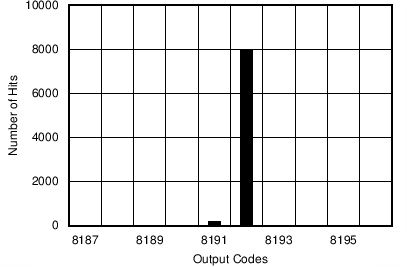Mean = 8192.5, sigma = 0.34, input = 0.625 × VREF, range = 1.25 × VREF
Figure 10. DC Histogram for Mid-Scale Inputs
(1.25 × VREF)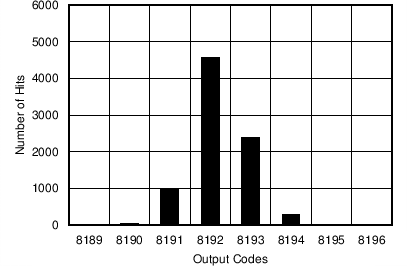Mean = 8192.5, sigma = 0.72, input = 0 V, range = ±0.15625 × VREF
Figure 12. DC Histogram for Mid-Scale Inputs
(±0.15625 x VREF)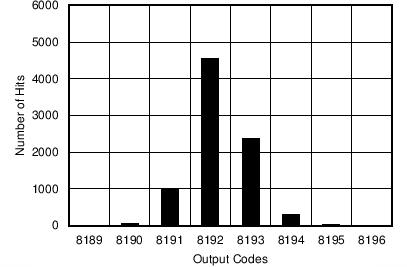Mean = 8192.5, sigma = 0.750.72, input = 0.15625 × VREF, range = 0.3125 × VREF
Figure 14. DC Histogram for Mid-Scale Inputs
(0.3125 x VREF)All input ranges
Figure 16. DNL vs TemperatureRange = ±1.25 × VREF
Figure 18. Typical INL for All Codes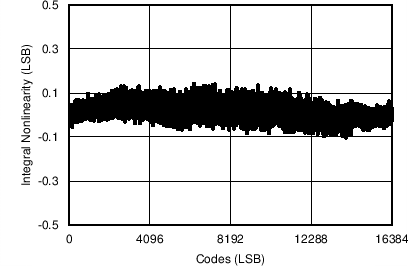Range = 2.5 × VREF
Figure 20. Typical INL for All Codes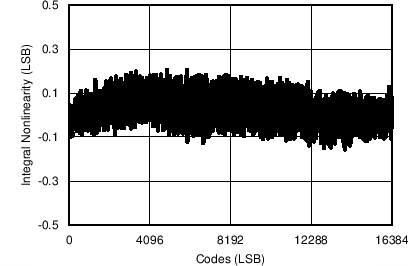Range = ±0.3125 × VREF
Figure 22. Typical INL for All Codes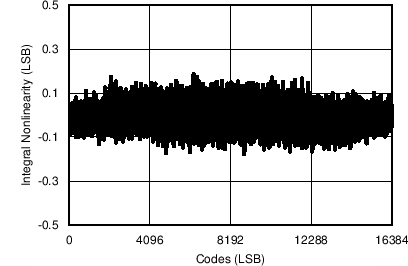Range = 0.625 × VREF
Figure 24. Typical INL for All Codes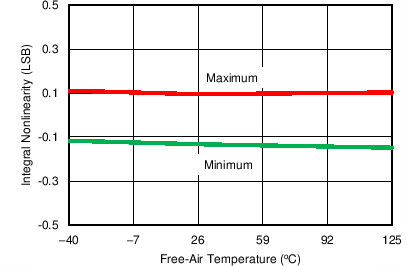Range = ±2.5 × VREF
Figure 26. INL vs Temperature (±2.5 × VREF)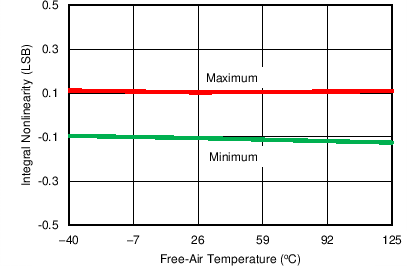Range = ±0.625 × VREF
Figure 28. INL vs Temperature (±0.625 × VREF)Range = 1.25 × VREF
Figure 30. INL vs Temperature (1.25 × VREF)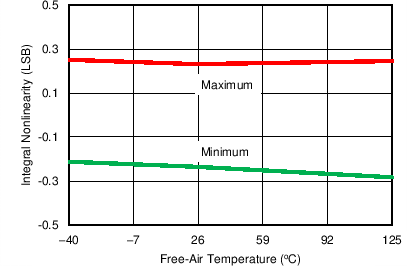Range = ±0.15625 × VREF
Figure 32. INL vs Temperature (±0.15625 × VREF)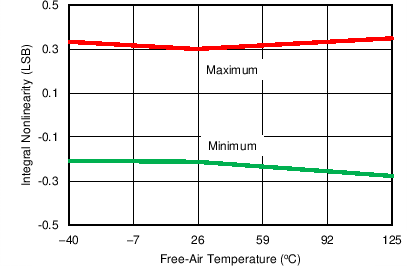Range = 0.3125 × VREF
Figure 34. INL vs Temperature (0.3125 × VREF)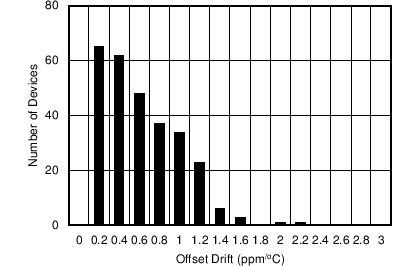Range = ±2.5 × VREF
Figure 36. Typical Histogram for Offset DriftFigure 38. Gain Error vs Temperature Across Input Ranges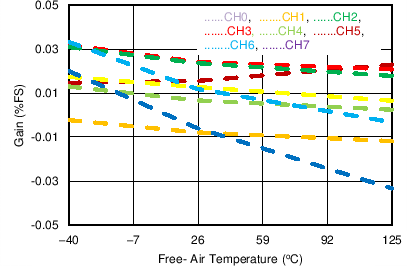Range = ±2.5 × VREF
Figure 40. Gain Error vs Temperature Across Channels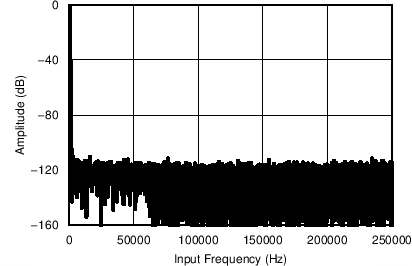Number of points = 16k, fIN = 1 kHz, SNR = 85.37 dB, SINAD = 85.35, THD = –104.68 dB, SFDR = 105 dB
Figure 42. Typical FFT Plot (±2.5 × VREF)Number of points = 16k, fIN = 1 kHz, SNR = 84.69 dB, SINAD = 84.67 dB, THD = –106.41 dB, SFDR = 105 dB
Figure 44. Typical FFT Plot (±0.625 × VREF)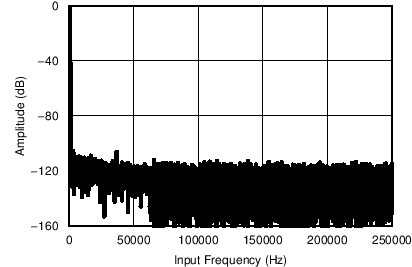Number of points = 16k, fIN = 1 kHz, SNR = 84.62 dB, SINAD = 84.65 dB, THD = –103.74 dB, SFDR = 105 dB
Figure 46. Typical FFT Plot (1.25 × VREF)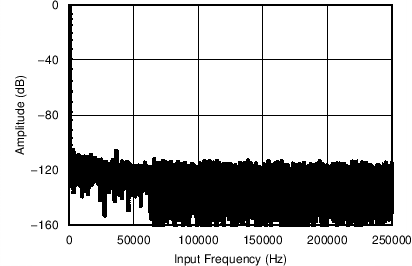Number of points = 16k, fIN = 1 kHz, SNR = 78.09 dB, SINAD = 78.06 dB, THD = –99.41 dB, SFDR = 105 dB
Figure 48. Typical FFT Plot (±0.15625 × VREF)Number of points = 16k, fIN = 1 kHz, SNR = 78.09 dB, SINAD = 78.05 dB, THD = –96.74 dB, SFDR = 105 dB
Figure 50. Typical FFT Plot (0.3125 × VREF)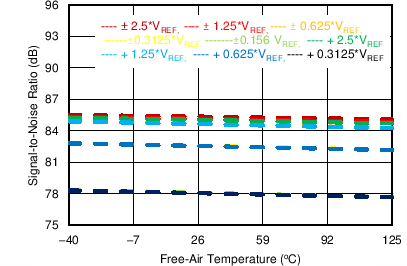fIN = 1 kHz
Figure 52. SNR vs Temperature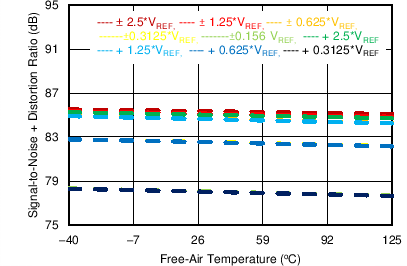fIN = 1 kHz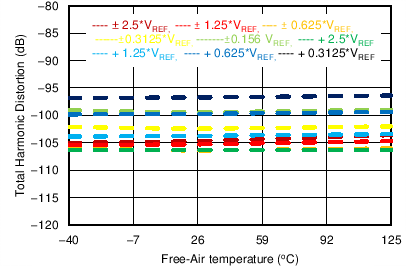fIN = 1 kHz
Figure 56. THD vs Temperature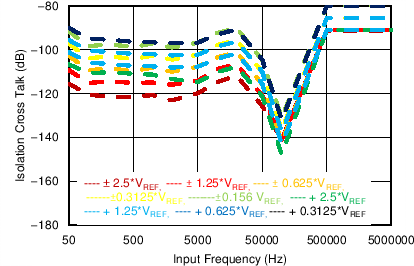Figure 58. Isolation Crosstalk vs Frequency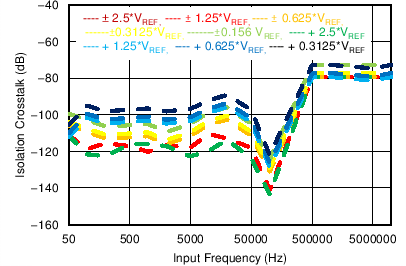Input = 2 × maximum input voltage
Figure 60. Isolation Crosstalk vs Frequency for
Overrange Inputs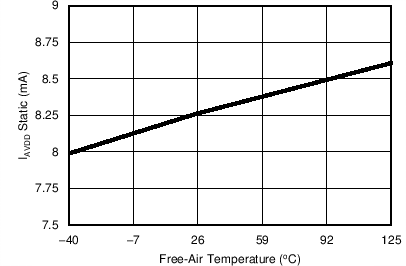Figure 62. AVDD Current vs Temperature for the ADS8678
(During Sampling)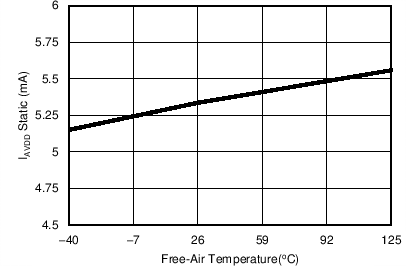Figure 64. AVDD Current vs Temperature for the ADS8674
(During Sampling)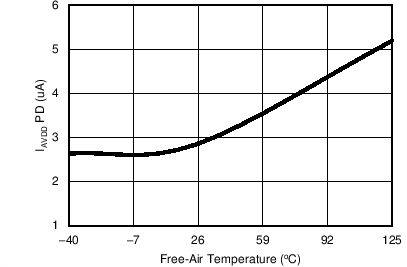Figure 66. AVDD Current vs Temperature
(Power Down)Input range = ±2.5 × VREF
Figure 3. Input Current vs Temperature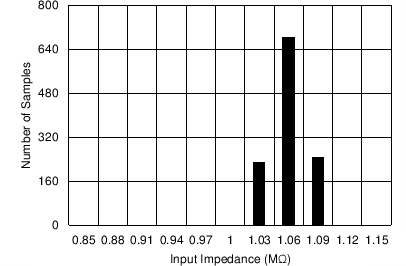Number of samples = 1160
Figure 5. Typical Distribution of Input Impedance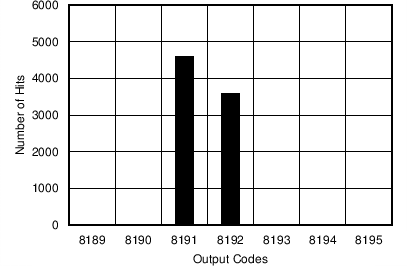Mean = 8192.5, sigma = 0.32, input = 0 V, range = ±1.25 × VREF
Figure 7. DC Histogram for Mid-Scale Inputs (±1.25 × VREF)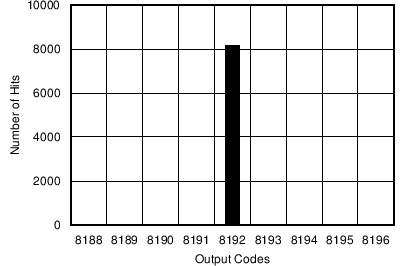Mean = 8192.5, sigma = 0.32, input = 1.25 × VREF, range = 2.5 × VREF
Figure 9. DC Histogram for Mid-Scale Inputs (2.5 × VREF)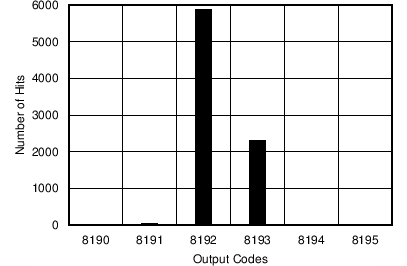Mean = 8192.5, sigma = 0.43, input = 0 V, range = ±0.3125 × VREF
Figure 11. DC Histogram for Mid-Scale Inputs
(±0.3125 x VREF)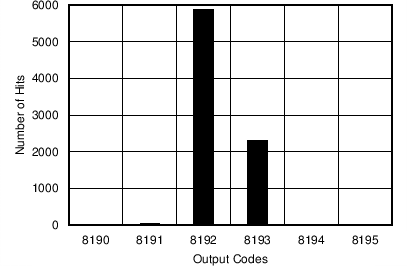Mean = 8192.5, sigma = 0.72, input = 0.3125 × VREF, range = 0.625 × VREF
Figure 13. DC Histogram for Mid-Scale Inputs
(0.625 x VREF)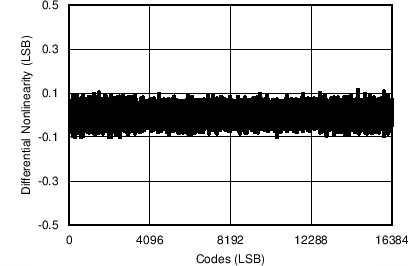All input ranges
Figure 15. Typical DNL for All Codes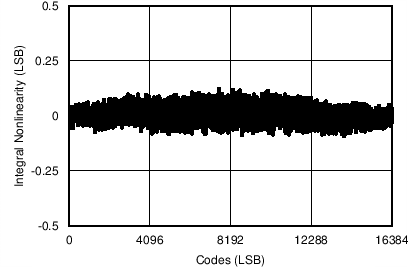Range = ±2.5 × VREF
Figure 17. Typical INL for All Codes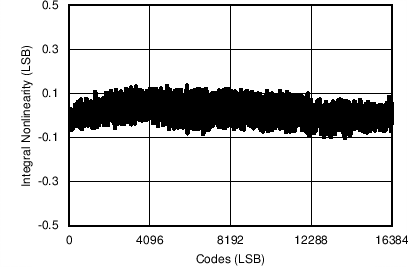Range = ±0.625 × VREF
Figure 19. Typical INL for All Codes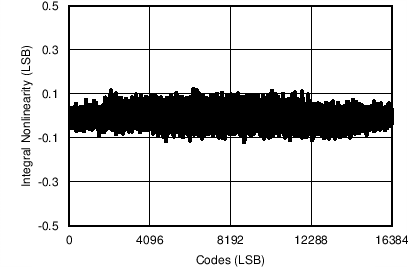Range = 1.25 × VREF
Figure 21. Typical INL for All Codes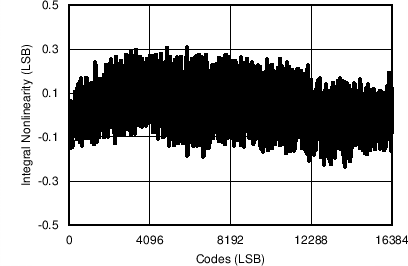Range = ±0.15625 × VREF
Figure 23. Typical INL for All Codes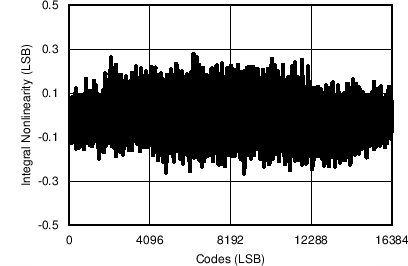Range = 0.3125 × VREF
Figure 25. Typical INL for All Codes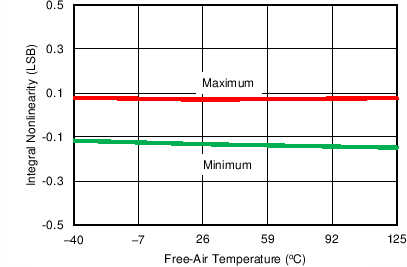Range = ±1.25 × VREF
Figure 27. INL vs Temperature (±1.25 × VREF)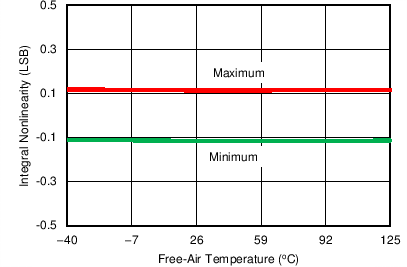Range = 2.5 × VREF
Figure 29. INL vs Temperature (2.5 × VREF)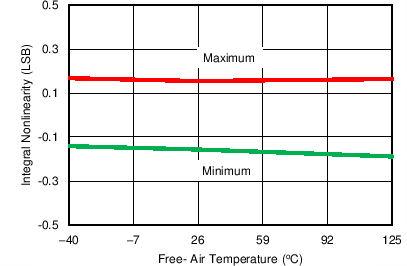Range = ±0.3125 × VREF
Figure 31. INL vs Temperature (±0.3125 × VREF)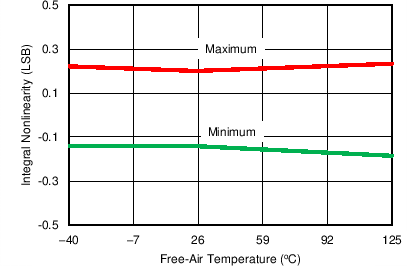Range = 0.625 × VREF
Figure 33. INL vs Temperature (0.625 × VREF)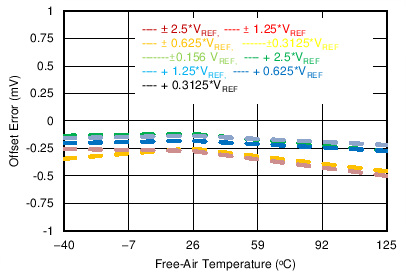Figure 35. Offset Error vs
Temperature Across Input Ranges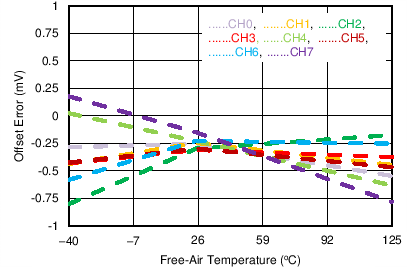Range = ±2.5 × VREF
Figure 37. Offset Error vs Temperature Across Channels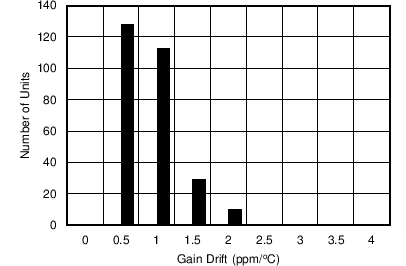Range = ±2.5 × VREF
Figure 39. Typical Histogram for Gain Error Drift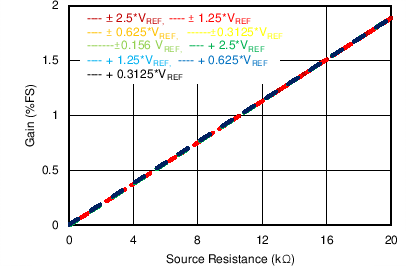Figure 41. Gain Error vs External Resistance (REXT)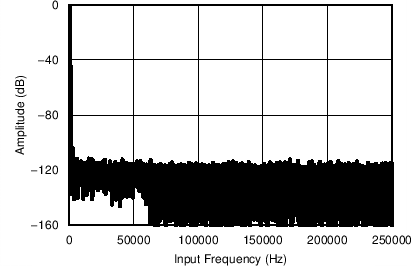Number of points = 16k, fIN = 1 kHz, SNR = 85.11 dB, SINAD = 85.15 dB, THD = –105.28 dB, SFDR = 105 dB
Figure 43. Typical FFT Plot (±1.25 × VREF)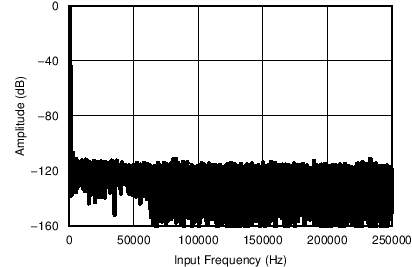Number of points = 16k, fIN = 1 kHz, SNR = 85.04 dB, SINAD = 85.04 dB, THD = –106.31 dB, SFDR = 105 dB
Figure 45. Typical FFT Plot (2.5 × VREF)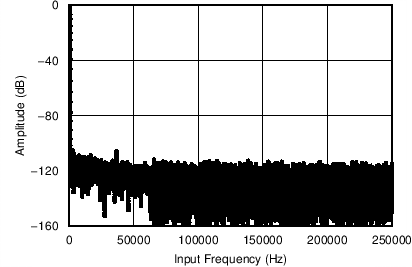Number of points = 16k, fIN = 1 kHz, SNR = 82.59 dB, SINAD = 82.56 dB, THD = –102.41 dB, SFDR = 105 dB
Figure 47. Typical FFT Plot (±0.3125 × VREF)Number of points = 16k, fIN = 1 kHz, SNR = 82.59 dB, SINAD = 82.55 dB, THD = –99.74 dB, SFDR = 105 dB
Figure 49. Typical FFT Plot (0.625 × VREF)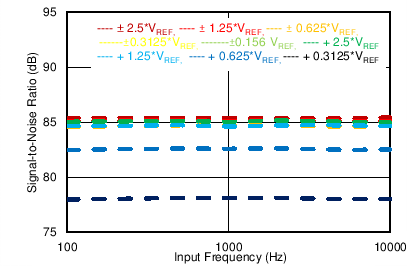Figure 51. SNR vs Input Frequency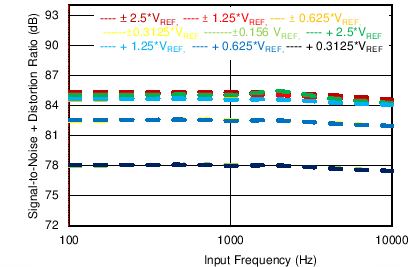Figure 53. SINAD vs Input Frequency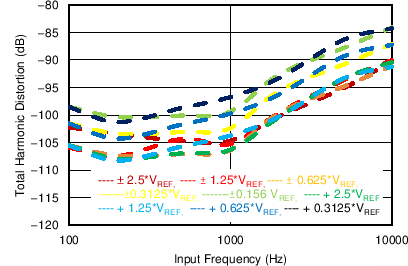Figure 55. THD vs Input Frequency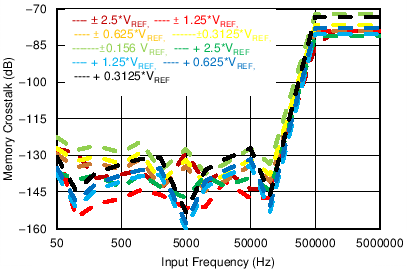Figure 57. Memory Crosstalk vs FrequencyInput = 2 × maximum input voltage
Figure 59. Memory Crosstalk vs Frequency for
Overrange Inputs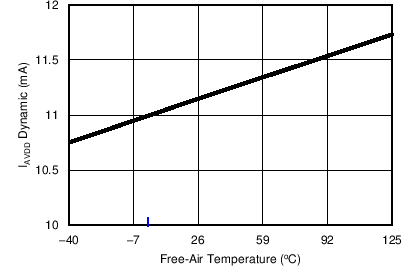Figure 61. AVDD Current vs Temperature for the ADS8678
(fS = 500 kSPS)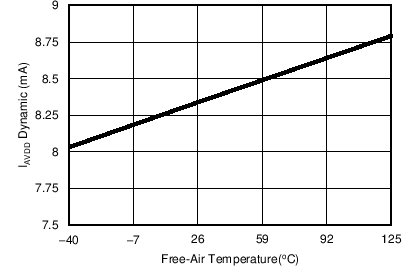Figure 63. AVDD Current vs Temperature for the ADS8674
(fS = 500 kSPS)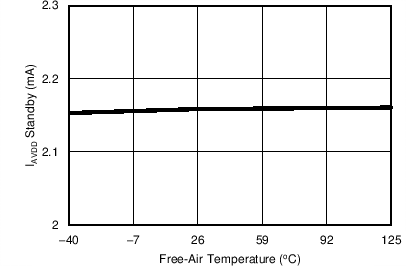Figure 65. AVDD Current vs Temperature
(STANDBY)